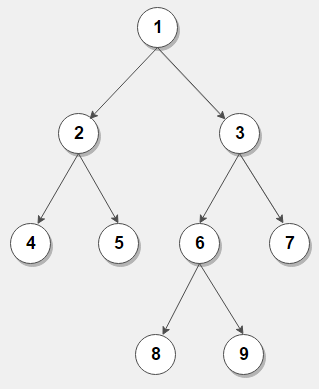# Print leaf to root path for every leaf node in a binary tree

Given a binary tree, write an iterative algorithm to print leaf to root path for every leaf node of binary tree. Use of Recursion is prohibited.

For example, consider below binary treeThere are five leaf to root path in above binary tree –

4 -> 2 -> 1
5 -> 2 -> 1
8 -> 6 -> 3 -> 1
9 -> 6 -> 3 -> 1
7 -> 3 -> 1

Since we’re not allowed to use recursion, we can do postorder iterative traversal of the tree and while doing so, we maintain a map that contains (child, parent) pair for every encountered node. Now if a leaf node is encountered, we can easily print leaf to root path using that map as shown below:

## C++

Output:

4 -> 2 -> 1
5 -> 2 -> 1
8 -> 6 -> 3 -> 1
9 -> 6 -> 3 -> 1
7 -> 3 -> 1

The time complexity of above solution is `O(nlog(n))` and needs extra `O(n)` space for the map. Unless we maintain a parent pointer in each of the tree nodes, the problem seems very difficult to solve without using any additional extra space apart from stack.

There is one workaround that doesn’t involve maintaining a parent pointer or use of any additional extra space. We can store path from root to leaf in a string as we traverse the tree iteratively and print the path whenever we encounter any leaf node.

## C++

Output:

4 -> 2 -> 1
5 -> 2 -> 1
8 -> 6 -> 3 -> 1
9 -> 6 -> 3 -> 1
7 -> 3 -> 1

Exercise:

1. Write recursive implementation of above problem.

2. Modify the solution to print leaf to root path having sum of nodes equal to a given number.(1 votes, average: 5.00 out of 5)Loading...